Publicité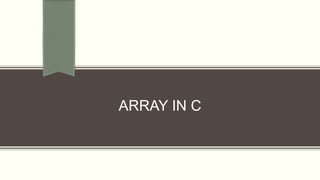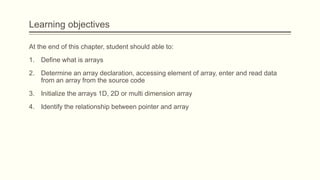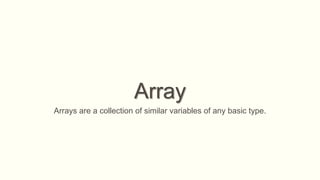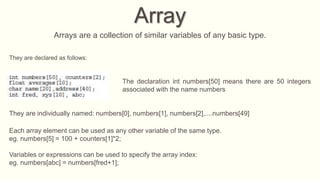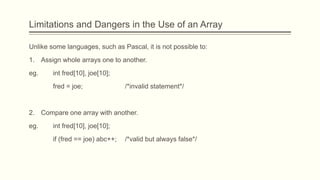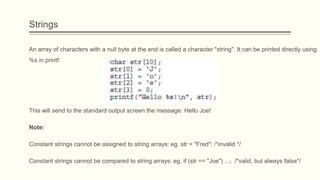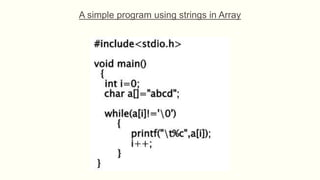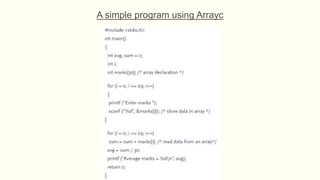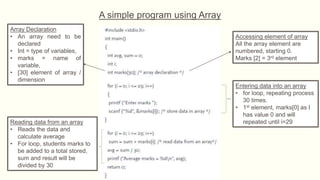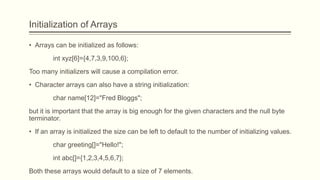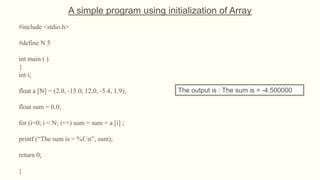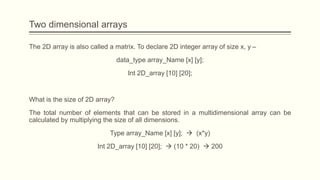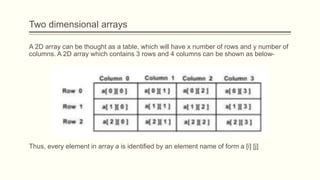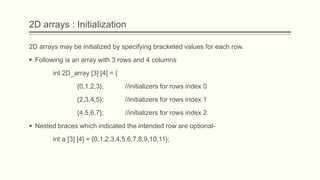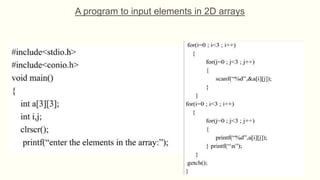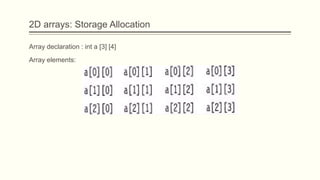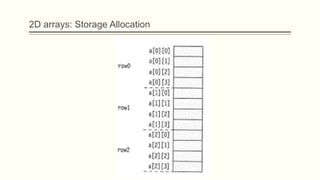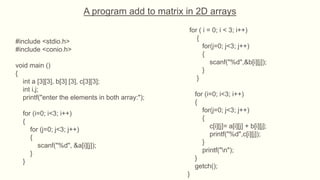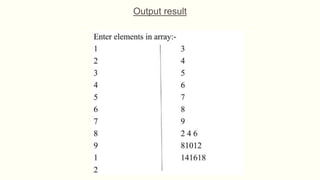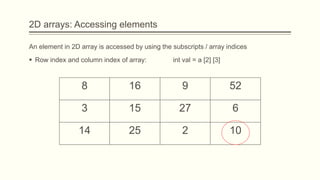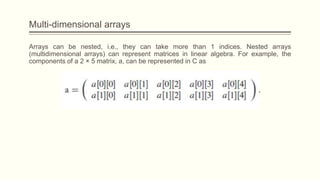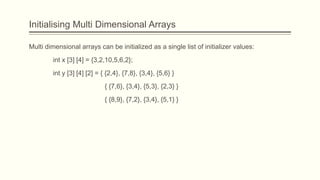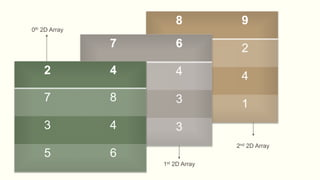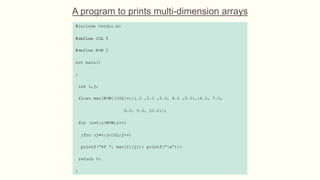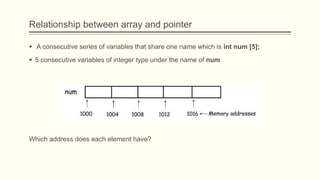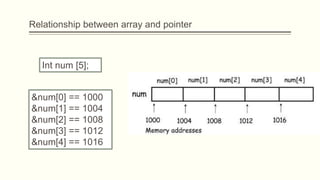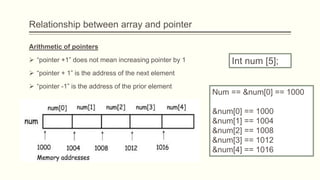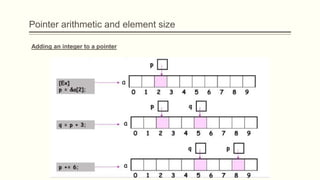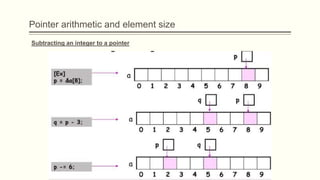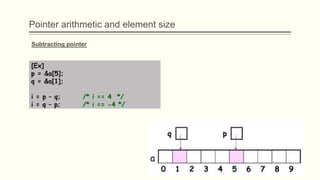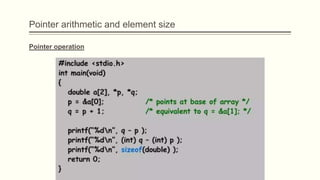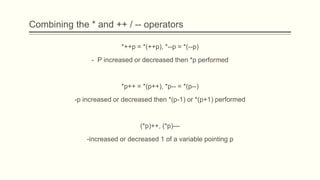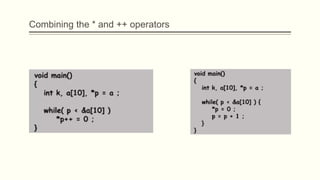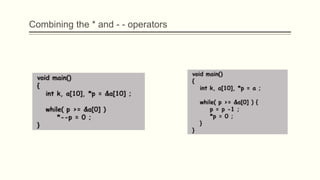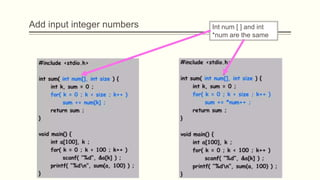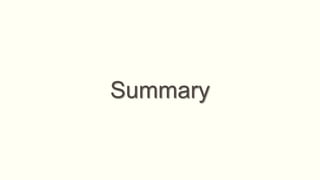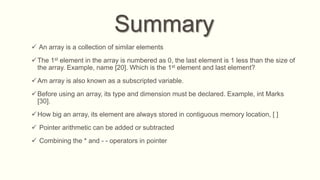1 sur 38
Publicité

### Chapter 13.pptx

1. ARRAY IN C
2. Learning objectives At the end of this chapter, student should able to: 1. Define what is arrays 2. Determine an array declaration, accessing element of array, enter and read data from an array from the source code 3. Initialize the arrays 1D, 2D or multi dimension array 4. Identify the relationship between pointer and array
3. Array Arrays are a collection of similar variables of any basic type.
4. Array Arrays are a collection of similar variables of any basic type. They are declared as follows: The declaration int numbers means there are 50 integers associated with the name numbers They are individually named: numbers, numbers, numbers,....numbers Each array element can be used as any other variable of the same type. eg. numbers = 100 + counters*2; Variables or expressions can be used to specify the array index: eg. numbers[abc] = numbers[fred+1];
5. Limitations and Dangers in the Use of an Array Unlike some languages, such as Pascal, it is not possible to: 1. Assign whole arrays one to another. eg. int fred, joe; fred = joe; /*invalid statement*/ 2. Compare one array with another. eg. int fred, joe; if (fred == joe) abc++; /*valid but always false*/
6. Strings An array of characters with a null byte at the end is called a character "string". It can be printed directly using %s in printf: This will send to the standard output screen the message: Hello Joe! Note: Constant strings cannot be assigned to string arrays: eg. str = "Fred"; /*invalid */ Constant strings cannot be compared to string arrays: eg. if (str == "Joe") ...; /*valid, but always false*/
7. A simple program using strings in Array
8. A simple program using Arrayc
9. A simple program using Array Array Declaration • An array need to be declared • Int = type of variables, • marks = name of variable, •  element of array / dimension Accessing element of array All the array element are numbered, starting 0. Marks  = 3rd element Entering data into an array • for loop, repeating process 30 times. • 1st element, marks as I has value 0 and will repeated until i=29 Reading data from an array • Reads the data and calculate average • For loop, students marks to be added to a total stored, sum and result will be divided by 30
10. Initialization of Arrays • Arrays can be initialized as follows: int xyz={4,7,3,9,100,6}; Too many initializers will cause a compilation error. • Character arrays can also have a string initialization: char name="Fred Bloggs"; but it is important that the array is big enough for the given characters and the null byte terminator. • If an array is initialized the size can be left to default to the number of initializing values. char greeting[]="Hello!"; int abc[]={1,2,3,4,5,6,7}; Both these arrays would default to a size of 7 elements.
11. #include <stdio.h> #define N 5 int main ( ) { int i; float a [N] = (2.0, -15.0, 12.0, -5.4, 1.9); float sum = 0.0; for (i=0; i < N; i++) sum = sum + a [i] ; printf (“The sum is = %f.n”, sum); return 0; } The output is : The sum is = -4.500000 A simple program using initialization of Array
12. Two dimensional arrays The 2D array is also called a matrix. To declare 2D integer array of size x, y – data_type array_Name [x] [y]; Int 2D_array  ; What is the size of 2D array? The total number of elements that can be stored in a multidimensional array can be calculated by multiplying the size of all dimensions. Type array_Name [x] [y];  (x*y) Int 2D_array  ;  (10 * 20)  200
13. A 2D array can be thought as a table, which will have x number of rows and y number of columns. A 2D array which contains 3 rows and 4 columns can be shown as below- Thus, every element in array a is identified by an element name of form a [i] [j] Two dimensional arrays
14. 2D arrays : Initialization 2D arrays may be initialized by specifying bracketed values for each row.  Following is an array with 3 rows and 4 columns int 2D_array   = { {0,1,2,3}; //initializers for rows index 0 {2,3,4,5}; //initializers for rows index 1 {4,5,6,7}; //initializers for rows index 2  Nested braces which indicated the intended row are optional- int a   = {0,1,2,3,4,5,6,7,8,9,10,11};
15. A program to input elements in 2D arrays
16. Output result
17. 2D arrays: Storage Allocation Array declaration : int a   Array elements:
18. 2D arrays: Storage Allocation
19. A program add to matrix in 2D arrays #include <stdio.h> #include <conio.h> void main () { int a , b , c; int i,j; printf("enter the elements in both array:"); for (i=0; i<3; i++) { for (j=0; j<3; j++) { scanf("%d", &a[i][j]); } } for ( i = 0; i < 3; i++) { for(j=0; j<3; j++) { scanf("%d",&b[i][j]); } } for (i=0; i<3; i++) { for(j=0; j<3; j++) { c[i][j]= a[i][j] + b[i][j]; printf("%d",c[i][j]); } printf("n"); } getch(); }
20. Output result
21. 2D arrays: Accessing elements An element in 2D array is accessed by using the subscripts / array indices  Row index and column index of array: int val = a   8 16 9 52 3 15 27 6 14 25 2 10
22. Multi-dimensional arrays Arrays can be nested, i.e., they can take more than 1 indices. Nested arrays (multidimensional arrays) can represent matrices in linear algebra. For example, the components of a 2 × 5 matrix, a, can be represented in C as
23. Initialising Multi Dimensional Arrays Multi dimensional arrays can be initialized as a single list of initializer values: int x   = {3,2,10,5,6,2}; int y    = { {2,4}, {7,8}, {3,4}, {5,6} } { {7,6}, {3,4}, {5,3}, {2,3} } { {8,9}, {7,2}, {3,4}, {5,1} }
24. 8 9 7 2 3 4 5 1 7 6 3 4 5 3 2 3 2 4 7 8 3 4 5 6 0th 2D Array 1st 2D Array 2nd 2D Array
25. A program to prints multi-dimension arrays
26. Relationship between array and pointer  A consecutive series of variables that share one name which is int num ;  5 consecutive variables of integer type under the name of num Which address does each element have?
27. Relationship between array and pointer Int num ; &num == 1000 &num == 1004 &num == 1008 &num == 1012 &num == 1016
28. Arithmetic of pointers  “pointer +1” does not mean increasing pointer by 1  “pointer + 1” is the address of the next element  “pointer -1” is the address of the prior element Relationship between array and pointer Int num ; Num == &num == 1000 &num == 1000 &num == 1004 &num == 1008 &num == 1012 &num == 1016
29. Pointer arithmetic and element size Adding an integer to a pointer
30. Pointer arithmetic and element size Subtracting an integer to a pointer
31. Pointer arithmetic and element size Subtracting pointer
32. Pointer operation Pointer arithmetic and element size
33. Combining the * and ++ / -- operators *++p = *(++p), *--p = *(--p) - P increased or decreased then *p performed *p++ = *(p++), *p-- = *(p--) -p increased or decreased then *(p-1) or *(p+1) performed (*p)++, (*p)— -increased or decreased 1 of a variable pointing p
34. Combining the * and ++ operators
35. Combining the * and - - operators
36. Add input integer numbers Int num [ ] and int *num are the same
37. Summary
38. Summary  An array is a collection of similar elements The 1st element in the array is numbered as 0, the last element is 1 less than the size of the array. Example, name . Which is the 1st element and last element? Am array is also known as a subscripted variable. Before using an array, its type and dimension must be declared. Example, int Marks . How big an array, its element are always stored in contiguous memory location, [ ]  Pointer arithmetic can be added or subtracted  Combining the * and - - operators in pointer

### Notes de l'éditeur

1. NOTE: To change the image on this slide, select the picture and delete it. Then click the Pictures icon in the placeholder to insert your own image.
Publicité